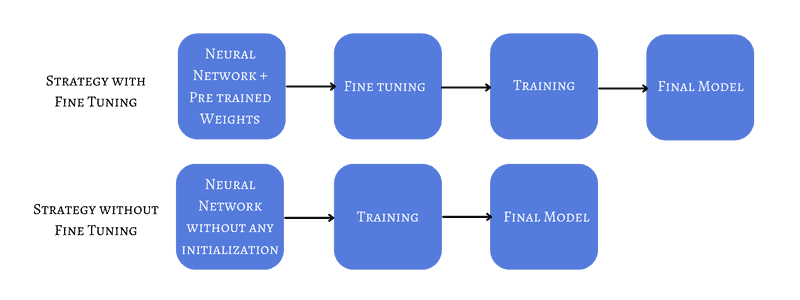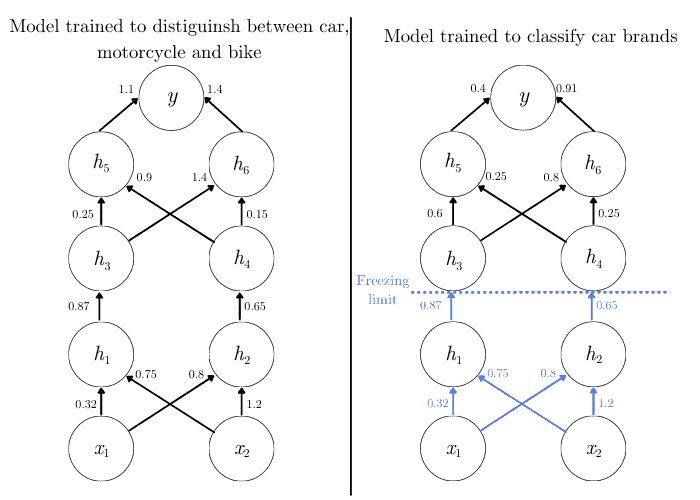## 1. Introduction

Nowadays, some convolutional neural network architectures such as GPipe have up to 557 million parameters. With our everyday computers, training such models would be nearly impossible considering computational costs.

In this tutorial, we’ll talk about fine-tuning, a way to overcome this issue.

## 2. What Is Fine-Tuning?

Before diving into its details, let’s first see why we need fine-tuning and what it is.

### 2.1. Motivation

Let’s suppose we want to train a model to detect cancer in human cells. To achieve good results, we decide to use a large deep neural network architecture.

Let’s suppose that our training dataset has only 1 000 samples, but that we have a network trained with 200 000 samples to classify images of human tissues and that it has many layers and neurons.

Instead of training a new network from scratch, we can re-use the existing one. So, we start the training on the new data but with the weights initialized to those of the tissue-classification network. That’s an example of fine-tuning.

If we didn’t use this approach, we would initially have random weights. In that case, a complex architecture would require a lot of time for backpropagation.

Besides, if we used our small dataset for a very deep network without a smart initialization strategy like the one above, we’d probably overfit the data.

### 2.2. Definition

In brief, fine-tuning refers to using the weights of an already trained network as the starting values for training a new network:The current best practices suggest using a model pre-trained with a large dataset for solving a problem similar to the one we’re dealing with. This is especially useful if we don’t have a lot of data for the task at hand, but a ready-to-use model for a related problem with a lot of data is available.

This can also lead to an overfit case if the chosen fine-tuning strategy is not appropriate.

## 3. Fine-tuning Strategies

We’ll talk about two different fine-tuning strategies. Usually, we combine them in practice.

### 3.1. Freezing Layers

The most common approach is to freeze the layers. What does that mean?

Naturally, we can initialize a neural network with pre-trained weights and let all weights be adjusted with the new training.

But, in this way, we might lose any learning ability.

One way to address the issue is to freeze only the first few layers and conduct the training for the others. Freezing the initial layer relies on the fact that in the initial stages, the network is learning basic features. This is exactly what we want to extract when we implement the fine-tuning. Alternatively, we can freeze all layers except the last one, whose weights we adjust during training.

Of course, we may need to change the output layer if, e.g., the old network was discriminating between two classes, but we have more classes in the current problem. The same goes for the first layer if the numbers of input features differ.

Finally, we can add several layers on top of the old architecture. That way, we keep all the old weights and change only the weights of new layers.

### 3.2. Example

As an example, let’s suppose we have two different tasks. The first one is a model trained with a large dataset to classify vehicles as cars, motorcycles, or bikes.

But, in our new application, we need to classify car brands for a small dataset. What we can do is freeze the weights connecting the first hidden layer (\$h_{1}\$ and \$h_{2}\$) and train the rest of the network.

In this way, we’ll only be able to update the weights of the subsequent layers. So, we keep the features learned by the initial layer and focus on the application-specific features in the other layers:However, let’s not forget about the last layer. If we previously had three classes, and now we have 35 car brands, we should update the architecture before training to reflect that.

### 3.3. Changing the Learning Rate

An implicit assumption of fine-tuning is that the datasets aren’t substantially different.

Because of that, we assume that the preceding training is useful. So, we don’t want to dramatically change the values of the weights.

One of the most important parameters that might affect the weights is the learning rate. This parameter defines how to update the weights.

Since we assume that we are using a good model, we should reduce the learning rate in our new training. That implies that the starting model should perform well on the new dataset.

For the learning rate during fine-tuning, we often use a value up to 10 times smaller than usual. As a result, our model will try to adapt itself to the new dataset in small steps. With this approach, we avoid losing the features it has already learned.

For instance, let’s assume that we trained a model to distinguish between dogs and cats. The earlier learning rate was equal to 0.01. But, now we’re interested in training a model to identify cat breeds. In that case, we can use the same neural network architecture and weights but adjust the learning rate to 0.001.

## 4. Shortcomings

Although we discussed the advantages of fine-tuning, it isn’t a magical solution that should always be used.

The first disadvantage is the domain-similarity requirement that should be satisfied. If that isn’t the case, there are no justifications for fine-tuning.

Moreover, we should also take into consideration that we won’t be able to change a single layer of the given architecture. That’s especially true if we want to keep all the existing weights. In turn, if we want to use our own architecture, fine-tuning isn’t an option.

Additionally, if our fine-tuning choices (which layers to freeze, what learning rate to use) are wrong, we may end up with a low-quality model. For instance, the weights can get trapped by a local minimum. In this scenario, our model never converges or acquires any learning ability.

Certainly, this is one of the main concerns in Machine Learning, not only when using fine-tuning. But, in this case, training from scratch (if we have a lot of data) would avoid such a problem.

## 5. Conclusion

In this article, we talked about fine-tuning. We should always consider it when we want to reduce the training time. Using the features learned in prior training will make our model converge faster.

By avoiding overfitting in small datasets, fine-tuning can help us achieve a model with satisfactory performance and good generalization capability.